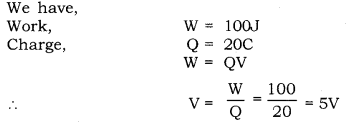# (a) Define the term Volt’

(a) Define the term Volt’.
(b) State the relation between work, charge and potential difference for an electric circuit. Calculate the potential difference between the two terminals of a battery if 100 of work is required to transfer 20 coulombs of charge from one terminal of the battery to the other.

a) Volt: Volt is the unit of potential difference between two points. If 1 joule work is done to move 1 coulomb positive charge from one point to another, then the potential difference between the points is 1 volt.
IV = 1JC-1
One volt is also defined as the potential difference across a resistance of 1 Ohm when 1 ampere of current flows through it.

(b) The relation between work (W), charge (Q) and potential difference (V): The work required to be done (W) to move a positive charge Q, between two points in a circuit having potential difference V, is QV. That is, W = QVTherefore, the potential difference between the two terminals of a battery is 5V.